UPSC  >  Important Formulae: Geometry & Mensuration

# Important Formulae: Geometry & Mensuration - CSAT Preparation - UPSC

### Lines and Angles

• Sum of the angles in a straight line is 180°
• Vertically opposite angles are congruent (equal).
• If any point is equidistant from the endpoints of a segment, then it must lie on the perpendicular bisector
• When two parallel lines are intersected by a transversal, corresponding angles are equal, alternate angles are equal and co-interior angles are supplementary. (All acute angles formed are equal to each other and all obtuse angles are equal to each other)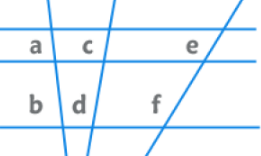EduRev's Tip: The ratio of intercepts formed by a transversal intersecting three parallel lines is equal to the ratio of corresponding intercepts formed by any other transversal.
⇒ a/b = c/d = e/f

### Triangles

• Sum of interior angles of a triangle is 180° and the sum of exterior angles is 360°.
• Exterior Angle = Sum of remote interior angles.
• Sum of two sides is always greater than the third side and the difference of two sides is always lesser than the third side.
• Side opposite to the biggest angle is longest and the side opposite to the smallest angle is the shortest.

Area of a triangle: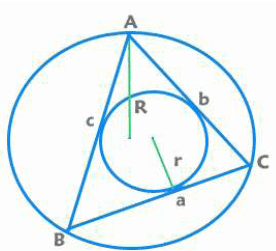= ½ x Base x Height
= ½ x Product of sides x Sine of included angle
=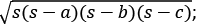here s is the semi perimeter [s = (a+b+c)/2 ]
= r x s [r is radius of incircle]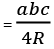[R is radius of circumcircle]

• A Median of a triangle is a line segment joining a vertex to the midpoint of the opposing side. The three medians intersect in a single point, called the Centroid of the triangle. Centroid divides the median in the ratio of 2:1.
• An Altitude of a triangle is a straight line through a vertex and perpendicular to the opposite side or an extension of the opposite side. The three altitudes intersect in a single point, called the Orthocenter of the triangle.
• A Perpendicular Bisector is a line that forms a right angle with one of the triangle's sides and intersects that side at its midpoint. The three perpendicular bisectors intersect in a single point, called the Circumcenter of the triangle. It is the center of the circumcircle which passes through all the vertices of the triangle.
• An Angle Bisector is a line that divides the angle at one of the vertices in two equal parts. The three angle bisectors intersect in a single point, called the Incenter of the triangle. It is the center of the incircle which touches all sides of a triangle.

EduRev's Tip:

• Centroid and Incenter will always lie inside the triangle.
(i) For an acute angled triangle, the Circumcenter and the Orthocenter will lie inside the triangle.
(ii) For an obtuse angled triangle, the Circumcenter and the Orthocenter will lie outside the triangle.
(iii) For a right angled triangle the Circumcenter will lie at the midpoint of the hypotenuse and the Orthocenter will lie at the vertex at which the angle is 90°.
• The orthocenter, centroid, and circumcenter always lie on the same line known as the Euler Line.
(i) The orthocenter is twice as far from the centroid as the circumcenter is.
(ii) If the triangle is Isosceles then the incenter lies on the same line.
(iii) If the triangle is equilateral, all four are the same point.

Theorems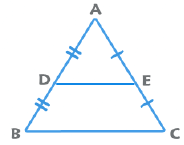Mid Point Theorem: The line joining the midpoint of any two sides is parallel to the third side and is half the length of the third side.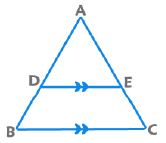Basic Proportionality Theorem: If DE || BC, then AD/DB = AE/EC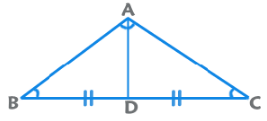Apollonius’ Theorem: AB2 + AC2 = 2 (AD2 + BD2)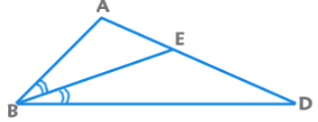Interior Angle Bisector Theorem: AE/ED = BA/BD

### Special Triangles

Right Angled Triangle:
∆ABC ≈ ∆ ADB ≈ ∆ BDC
BD2 = AD x DC and AB x BC = BD X DC

Equilateral Triangle: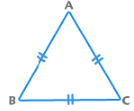All angles are equal to 60°. All sides are equal also.
Height =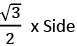Area =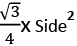Isosceles Triangle: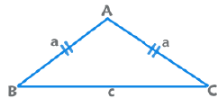Angles equal to opposite sides are equal.
Area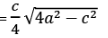30°-60°-90° Triangle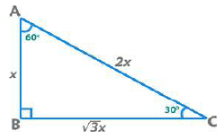Area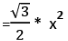45°-45°-90° Triangle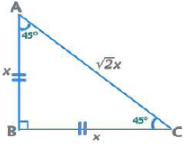Area = x2/2
30°-30°-120° Triangle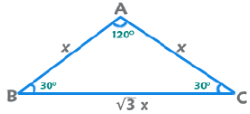Area =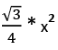Similarity of Triangles
Two triangles are similar if their corresponding angles are congruent and corresponding sides are in proportion.
Tests of similarity: (AA / SSS / SAS)
For similar triangles, if the sides are in the ratio of a:b
⇒ Corresponding heights are in the ratio of a:b
⇒ Corresponding medians are in the ratio of a:b
⇒ Circumradii are in the ratio of a:b
⇒ Inradii are in the ratio of a:b
⇒ Perimeters are in the ratio of a:b
⇒ Areas are in the ratio a2 : b2

Congruency of Triangles
Two triangles are congruent if their corresponding sides and angles are congruent.

Tests of congruence: (SSS / SAS / AAS / ASA)
All ratios mentioned in similar triangle are now 1:1

Polygons
Sum of interior angles = (n-2) x 180° = (2n-4) x  90° Sum of exterior angles = 360°
Number of diagonals =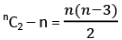Number of triangles which can be formed by the vertices = nC3

Regular Polygon:
If all sides and all angles are equal, it is a regular polygon. All regular polygons can be inscribed in or circumscribed about a circle.
Area = ½ x Perimeter x Inradius {Inradius is the perpendicular from centre to any side}
Each Interior Angle =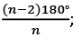Exterior = 360°/n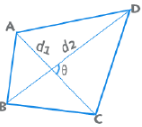Sum of the interior angles = Sum of the exterior angles = 360°
Area for a quadrilateral is given by ½ d1 d2 Sinθ.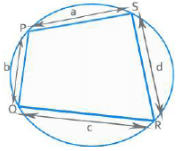If all vertices of a quadrilateral lie on the circumference of a circle, it is known as a cyclic quadrilateral.
Opposite angles are supplementary
Area =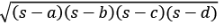where s is the semi perimeter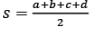EduRev's Tip:

• Sum or product of opposite sides = Product of diagonals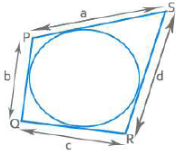• If a circle can be inscribed in a quadrilateral, its area is given by = √abcd

Parallelogram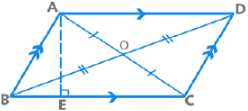• Opposite sides are parallel and congruent.
• Opposite angles are congruent and consecutive angles are supplementary.
• Diagonals of a parallelogram bisect each other.
• Perimeter = 2(Sum of adjacent sides);
• Area = Base x Height = AD x BE

EduRev's Tip:

• A parallelogram inscribed in a circle is always a Rectangle. A parallelogram circumscribed about a circle is always a Rhombus.
• Each diagonal divides a parallelogram in two triangles of equal area.
• Sum of squares of diagonals = Sum of squares of four sides
⇒ AC2 + BD2 = AB2 + BC2 + CD2 + DA2
• A Rectangle is formed by intersection of the four angle bisectors of a parallelogram.

Rhombus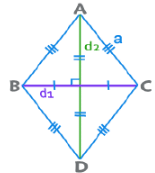A parallelogram with all sides equal is a Rhombus. Its diagonals bisect at 90°.
Perimeter = 4a;   Area = ½ d1 d2 ;   Area = d x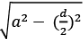Rectangle: A parallelogram with all angles equal (90°) is a Rectangle. Its diagonals are congruent. Perimeter = 2(l+b); Area = lb

Square: A parallelogram with sides equal and all angles equal is a square. Its diagonals are congruent and bisect at 90°.
Perimeter = 4a; Area = a2; Diagonals = a√2

EduRev's Tip: From all quadrilaterals with a given area, the square has the least perimeter. For all quadrilaterals with a given perimeter, the square has the greatest area.

Kite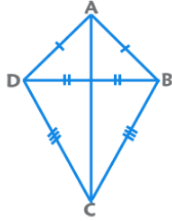• Two pairs of adjacent sides are congruent.
• The longer diagonal bisects the shorter diagonal at 90°.
• Area = Product of Diagonals / 2

Trapezium / Trapezoid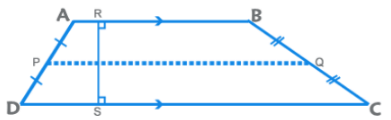A quadrilateral with exactly one pair of sides parallel is known as a Trapezoid. The parallel sides are known as bases and the non-parallel sides are known as lateral sides.
Area = ½ x (Sum of parallel sides) x Height
Median, the line joining the midpoints of lateral sides, is half the sum of parallel sides.

EduRev's Tip: Sum of the squares of the length of the diagonals = Sum of squares of lateral sides + 2 Product of bases.
⇒ AC2 + BD2 = AD2 + BC2 + 2 x AB x CD

Isosceles Trapezium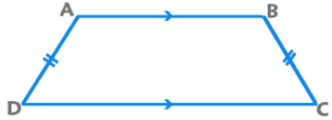The non-parallel sides (lateral sides) are equal in length. Angles made by each parallel side with the lateral sides are equal.
EduRev's Tip: If a trapezium is inscribed in a circle, it has to be an isosceles trapezium. If a circle can be inscribed in a trapezium, Sum of parallel sides = Sum of lateral sides.

Hexagon (Regular)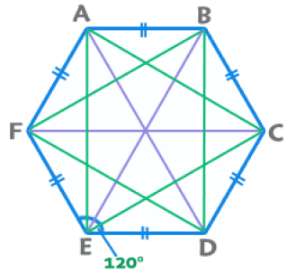Perimeter = 6a; Area =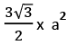Sum of Interior angles = 720°.
Each Interior Angle = 120°. Exterior = 60°
Number of diagonals = 9 {3 big and 6 small}
Length of big diagonals (3) = 2a
Length of small diagonals (6) = √3a

EduRev's Tip: A regular hexagon can be considered as a combination of six equilateral triangles. All regular polygons can be considered as a combination of ‘n’ isosceles triangles.
Area of a Pentagon = 1.72 a2
Area of an Octagon = 2(√2 + 1) a2

Circles
Diameter = 2r; Circumference = 2πr; Area = πr2
Chords equidistant from the centre of a circle are equal.
A line from the centre, perpendicular to a chord, bisects the chord.
Equal chords subtend equal angles at the centre.
The diameter is the longest chord of a circle.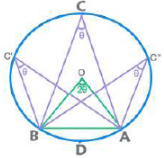A chord /arc subtends equal angle at any point on the circumference and double of that at the centre.
Chords / Arcs of equal lengths subtend equal angles.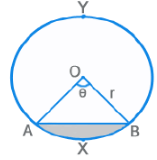Chord AB divides the circle into two parts: Minor Arc AXB and Major Arc AYB.
Measure of arc AXB = ∠AOB = θ
Length (arc AXB) =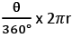Area (sector OAXB) =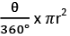Area of Minor Segment = Shaded Area in above figure
⇒ Area of Sector OAXB  - Area of ∆ OAB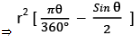Properties of Tangents, Secants and Chords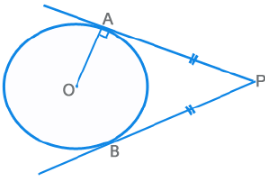The radius and tangent are perpendicular to each other.
There can only be two tangents from an external point, which are equal in length  PA = PB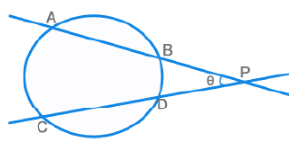PA x PB = PC x PD
θ = ½ [ m(Arc AC) – m(Arc BD) ]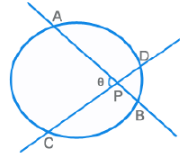PA x PB = PC x PD
θ = ½ [ m(Arc AC) + m(Arc BD) ]

Properties (contd.)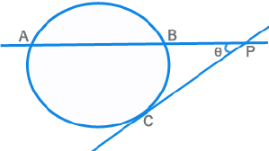PA x PB = PC2
θ = ½ [ m(Arc AC) - m(Arc BC) ]

Alternate Segment Theorem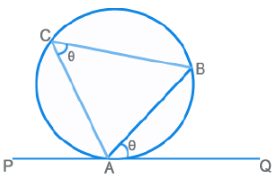The angle made by the chord AB with the tangent at A (PQ) is equal to the angle that it subtends on the opposite side of the circumference.
⇒ ∠BAQ = ∠ACB

Common Tangents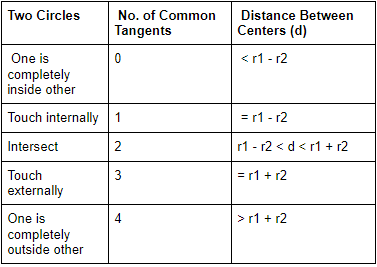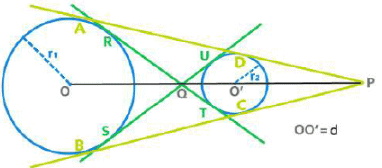⇒ AD = BC  =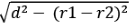Length of the Transverse Common Tangent (TCT)
⇒ RT = SU =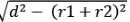EduRev's Tip:

• The two centers(O and O’), point of intersection of DCTs (P)and point of intersection of TCTs (Q) are collinear.  Q divides OO’ in the ratio r1 : r2 internally whearea P divides OO’ in the ratio r1 : r2 externally.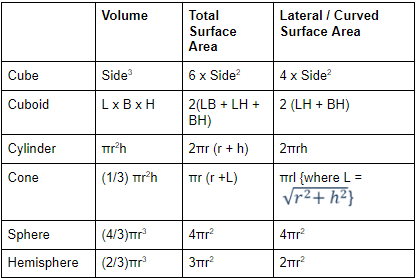• There are 4 body diagonals in a cube / cuboid of length (√3 x side) and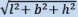respectively.
The document Important Formulae: Geometry & Mensuration | CSAT Preparation - UPSC is a part of the UPSC Course CSAT Preparation.
All you need of UPSC at this link: UPSC

## CSAT Preparation

197 videos|151 docs|200 tests

## FAQs on Important Formulae: Geometry & Mensuration - CSAT Preparation - UPSC

 1. What is the formula for finding the area of a rectangle?Ans. The formula for finding the area of a rectangle is length multiplied by width. Mathematically, it can be expressed as Area = Length x Width.
 2. How do you calculate the circumference of a circle?Ans. The circumference of a circle can be calculated using the formula 2πr, where r represents the radius of the circle. Alternatively, it can also be calculated using the formula πd, where d represents the diameter of the circle.
 3. What is the formula for finding the volume of a cylinder?Ans. The formula for finding the volume of a cylinder is given by V = πr^2h, where r represents the radius of the base and h represents the height of the cylinder.
 4. How do you calculate the perimeter of a triangle?Ans. The perimeter of a triangle is calculated by adding the lengths of all its sides. For example, if the lengths of the three sides of a triangle are a, b, and c, then the perimeter is given by P = a + b + c.
 5. What is the formula for finding the area of a circle?Ans. The formula for finding the area of a circle is A = πr^2, where r represents the radius of the circle.

## CSAT Preparation

197 videos|151 docs|200 testsExplore Courses for UPSC exam### How to Prepare for UPSC

Read our guide to prepare for UPSC which is created by Toppers & the best Teachers
Signup to see your scores go up within 7 days! Learn & Practice with 1000+ FREE Notes, Videos & Tests.
10M+ students study on EduRev
Track your progress, build streaks, highlight & save important lessons and more!
Related Searches

,

,

,

,

,

,

,

,

,

,

,

,

,

,

,

,

,

,

,

,

,

;